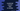# How to convert date to number in JavaScript## How to convert date to number in JavaScript:

In this post, we will learn how to convert a date to number in JavaScript. Date class provides a method called getTime, that can be used to convert a date to number.

In this post, I will show you how to use this with an example.

### Definition of getTime method:

getTime() method returns the number of milliseconds since Epoch or since 1 January 1970 00:00:00 UTC. It is a number.

``date.getTime()``

The advantage of getTime is that it works in UTC. So, it will always return the same result even if it is called from different timezones.

### Example of getTime:

Let’s take an example of how getTime works:

``````const time = new Date('July 30, 99 10:11:10 GMT+00:00');

console.log('time: ',time.getTime());``````

It will print:

``time:  933329470000``

It will always print 933329470000 irrespective of the timezone.

### Use getTime to find the execution time:

We can also use getTime to find the execution time. For example:

``````const startTime = new Date();

for(var i = 0; i< 1000000; i++){
Math.random(i);
}
const endTime = new Date();

console.log(`Time taken: \${endTime.getTime() - startTime.getTime()}msec`);``````

In this example, we are calculating the Date object before and after the for loop executed. The time taken is is calculated in milliseconds by finding the difference of getTime() for endTime and startTime.

It will print something like below:

``Time taken: 17msec``

It will be different in a different machine.